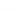• 作者

# * 夜伴M音～～Ｍ*Ｐ*３*

2006-09-29 00:14

＝＝＝＝＝＝＝

1 .

2 .

3 .

4 .

5

620)this.style.width=620" vspace=5 border=0>620)this.style.width=620" vspace=5 border=0>620)this.style.width=620" vspace=5 border=0>620)this.style.width=620" vspace=5 border=0>620)this.style.width=620" vspace=5 border=0>620)this.style.width=620" vspace=5 border=0>620)this.style.width=620" vspace=5 border=0>620)this.style.width=620" vspace=5 border=0>620)this.style.width=620" vspace=5 border=0>

﻿